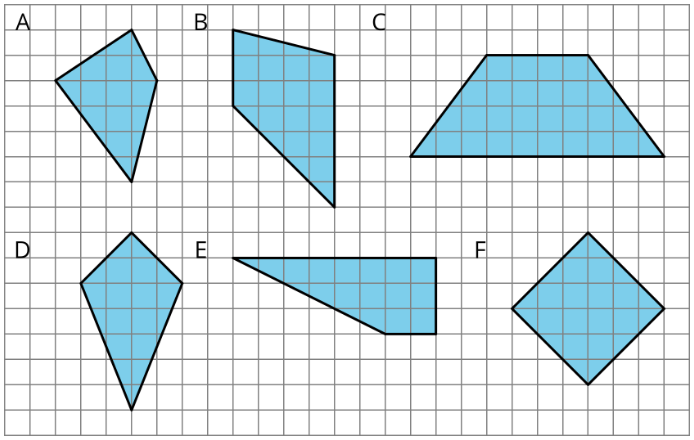$$\newcommand{\id}{\mathrm{id}}$$ $$\newcommand{\Span}{\mathrm{span}}$$ $$\newcommand{\kernel}{\mathrm{null}\,}$$ $$\newcommand{\range}{\mathrm{range}\,}$$ $$\newcommand{\RealPart}{\mathrm{Re}}$$ $$\newcommand{\ImaginaryPart}{\mathrm{Im}}$$ $$\newcommand{\Argument}{\mathrm{Arg}}$$ $$\newcommand{\norm}{\| #1 \|}$$ $$\newcommand{\inner}{\langle #1, #2 \rangle}$$ $$\newcommand{\Span}{\mathrm{span}}$$

# 1.4.1: Polygons

•• Contributed by Illustrative Mathematics
• OpenUp Resources
$$\newcommand{\vecs}{\overset { \rightharpoonup} {\mathbf{#1}} }$$ $$\newcommand{\vecd}{\overset{-\!-\!\rightharpoonup}{\vphantom{a}\smash {#1}}}$$$$\newcommand{\id}{\mathrm{id}}$$ $$\newcommand{\Span}{\mathrm{span}}$$ $$\newcommand{\kernel}{\mathrm{null}\,}$$ $$\newcommand{\range}{\mathrm{range}\,}$$ $$\newcommand{\RealPart}{\mathrm{Re}}$$ $$\newcommand{\ImaginaryPart}{\mathrm{Im}}$$ $$\newcommand{\Argument}{\mathrm{Arg}}$$ $$\newcommand{\norm}{\| #1 \|}$$ $$\newcommand{\inner}{\langle #1, #2 \rangle}$$ $$\newcommand{\Span}{\mathrm{span}}$$ $$\newcommand{\id}{\mathrm{id}}$$ $$\newcommand{\Span}{\mathrm{span}}$$ $$\newcommand{\kernel}{\mathrm{null}\,}$$ $$\newcommand{\range}{\mathrm{range}\,}$$ $$\newcommand{\RealPart}{\mathrm{Re}}$$ $$\newcommand{\ImaginaryPart}{\mathrm{Im}}$$ $$\newcommand{\Argument}{\mathrm{Arg}}$$ $$\newcommand{\norm}{\| #1 \|}$$ $$\newcommand{\inner}{\langle #1, #2 \rangle}$$ $$\newcommand{\Span}{\mathrm{span}}$$

## Lesson

Let's investigate polygons and their areas.

Exercise $$\PageIndex{1}$$: Which One Doesn't Belong: Bases and Heights

Which one doesn't belong?Figure $$\PageIndex{1}$$

Exercise $$\PageIndex{2}$$: What Are Polygons?

Here are five polygons:Figure $$\PageIndex{2}$$

Here are six figures that are not polygons:Figure $$\PageIndex{3}$$
1. Circle the figures that are polygons.Figure $$\PageIndex{4}$$
1. What do the figures you circled have in common? What characteristics helped you decide whether a figure was a polygon?

Exercise $$\PageIndex{3}$$: Quadrilateral StrategiesFigure $$\PageIndex{5}$$

Here is a trapezoid. $$a$$ and $$b$$ represent the lengths of its bottom and top sides. The segment labeled $$h$$ represents its height; it is perpendicular to both the top and bottom sides.Figure $$\PageIndex{6}$$

Apply area-reasoning strategies—decomposing, rearranging, duplicating, etc.—on the trapezoid so that you have one or more shapes with areas that you already know how to find. Use the shapes to help you write a formula for the area of a trapezoid. Show your reasoning.

Exercise $$\PageIndex{4}$$: Pinwheel

Find the area of the shaded region in square units. Show your reasoning.Figure $$\PageIndex{7}$$

### Summary

A polygon is a two-dimensional figure composed of straight line segments.

• Each end of a line segment connects to one other line segment. The point where two segments connect is a vertex. The plural of vertex is vertices.
• The segments are called the edges or sides of the polygon. The sides never cross each other. There are always an equal number of vertices and sides.

Here is a polygon with 5 sides. The vertices are labeled $$A, B, C, D$$, and $$E$$.Figure $$\PageIndex{8}$$

A polygon encloses a region. To find the area of a polygon is to find the area of the region inside it.

We can find the area of a polygon by decomposing the region inside it into triangles and rectangles.Figure $$\PageIndex{9}$$

The first two diagrams show the polygon decomposed into triangles and rectangles; the sum of their areas is the area of the polygon. The last diagram shows the polygon enclosed with a rectangle; subtracting the areas of the triangles from the area of the rectangle gives us the area of the polygon.

### Glossary Entries

Definition: Polygon

A polygon is a closed, two-dimensional shape with straight sides that do not cross each other.

Figure $$ABCDE$$ is an example of a polygon.Figure $$\PageIndex{10}$$

## Practice

Exercise $$\PageIndex{5}$$

Select all the polygons.Figure $$\PageIndex{11}$$
1. A
2. B
3. C
4. D
5. E
6. F

Exercise $$\PageIndex{6}$$

Mark each vertex with a large dot. How many edges and vertices does this polygon have?Figure $$\PageIndex{12}$$

Exercise $$\PageIndex{7}$$

Find the area of this trapezoid. Explain or show your strategy.Figure $$\PageIndex{13}$$

Exercise $$\PageIndex{8}$$

Lin and Andre used different methods to find the area of a regular hexagon with 6-inch sides. Lin decomposed the hexagon into six identical, equilateral triangles. Andre decomposed the hexagon into a rectangle and two triangles.Figure $$\PageIndex{14}$$: 2 identical hexagons labeled Lin's method and Andre's method. Each hexagon has three sides labeled 6 inches and a height labeled 10.4 inches. Lin's method, hexagon divided into 6 identical, equilateral triangles. Andre's method, hexagon decomposed into a rectangle and a triangle on either side of the rectangle.

Find the area of the hexagon using each person’s method. Show your reasoning.

Exercise $$\PageIndex{9}$$

1. Identify a base and a corresponding height that can be used to find the area of this triangle. Label the base $$b$$ and the corresponding height $$h$$.Figure $$\PageIndex{15}$$: A triangle on a grid that has two vertices 11 units apart from one another horizontally. The third vertex is 2 units below the horizontal side, 5 units to the right of the left vertex, and 6 units to the left of the right vertex.
1. Find the area of the triangle. Show your reasoning.

(From Unit 1.3.3)

Exercise $$\PageIndex{10}$$

On the grid, draw three different triangles with an area of 8 square units. Label the base and height of each triangle.Figure $$\PageIndex{16}$$

(From Unit 1.3.4)